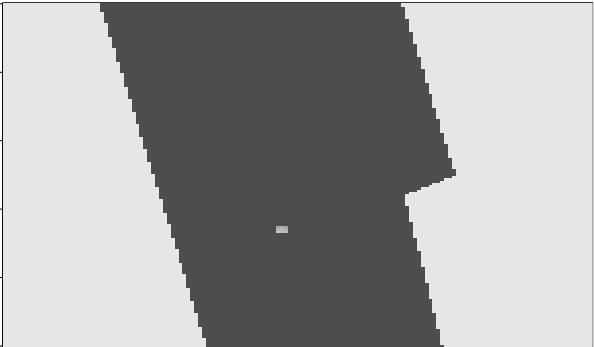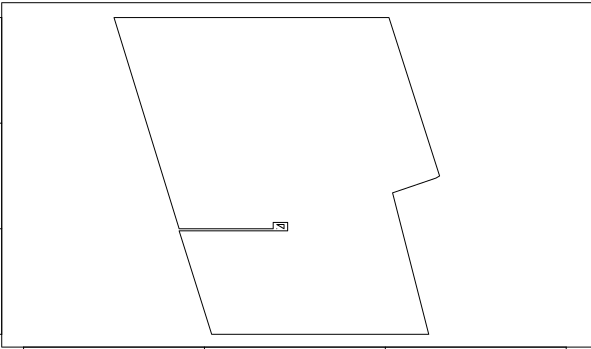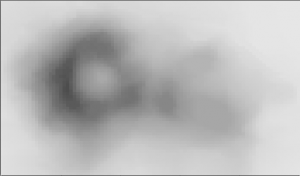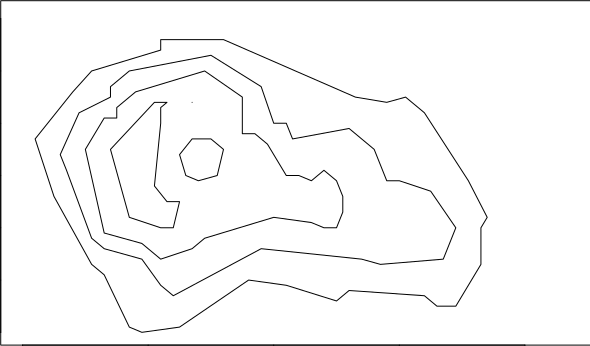# Vectorization of Raster

We would never start writing any vectorization code if there was any free library. However, recently Andy Bosyi was involved as a tech lead in an interesting project related to geometry and Vectorization of raster. We needed to calculate complex projections of numerous shapes. Since we started with a “clean” mathematical solution, we quickly ended up with a huge number of calculations related to polygon triangulation – O(log n!). It took us a week to figure out that we were in real trouble – for real scenarios the process lasted for minutes. Then we decided to turn our eyes towards discretization (as it was declared at the beginning) and we did the job and got the result in the form of a matrix. It was Friday and the results needed to be presented the next Monday. But in a vector form.

Our search for raster vectorization in the R packages revealed this package and since the function rasterToPolygons looked good, we thought perhaps it was producing too many points for the polygons. Since there was no R package which was necessary, we needed to create our own. Well, still having a half of the weekend, we did some C++ coding and created this function which does the job in one move. Here is the initial bitmap with an enclave and an option to not allow exclaves and the result:Example from the volcano dataset:
```library(fasteraster);
library(datasets);
inp = volcano; res = raster2vector(volcano, 120, 200, 20);
image(inp, col = rev(grey.colors(100)), useRaster = TRUE)
plot(0, type = "l", xlim = c(0, nrow(inp)), ylim = c(0, ncol(inp)))
a = lapply(res, function(x) lines(rbind(x, x[1,])))```
Produces:You can find source package: fasteraster_1.0.4.tar And Linux 64 binary here: fasteraster_1.0.4_R_x86_64-pc-linux-gnu.tar  Fresh version right from CRAN: https://cran.r-project.org/package=fasteraster Author: Andy Bosyi, CEO/Lead Data Scientist MindCraft Information Technology & Data Science